# 基于三步优化的多目标水资源配置研究及应用Multi-Objective Water Resources Allocation Based on Three-Step Method

• 全文下载: PDF(1809KB)    PP.433-444   DOI: 10.12677/JWRR.2018.75049
• 下载量: 195  浏览量: 397   国家自然科学基金支持

A multi-objective water resources optimal allocation model for transboundary region is established after an overall consideration of national economic water demand, water balance and eco-environmental water consumption. In order to ensure the equality among stakeholders, the index and benefit ratio is introduced, and the multi-objective problem is transformed into several single-objective problems by adopting the three-step method, which can greatly simplify the solution process. The proposed model and method are verified through the case study in the middle and lower reaches of Han River. The outputs of Highest Water Profit (HWP) model show that the allocation benefits of subdivisions located in Tangbai River basin have more effects on each other while the cities that are located downstream of Danjiangkou Reservoir do not depend upon the allocated water to others. The Highest Ratio of Highest Water Profit (HRHWP) model presents that the benefit ratio is lowest in summer, mainly due to the largest water demand in this period. In spring, autumn and winter, the indices all exceed 0.9. The results of Final Water Profit (FWP) model show that the water shortage rates are 3.11%, 3.69% and 3.94% at frequency of 50%, 75% and 95%, respectively. The domestic water consumption is basically satisfied at the planning year, but there are relatively larger shortages in agricultural irrigation and ecological environment water consumption. The water shortage in Tangbai River basin is more serious than that in the main stream area below Danjiangkou Reservoir.

1. 引言

2. 研究方法

2.1. 水资源优化配置模型

$\mathrm{max}\text{}{Z}_{k}=\underset{t}{\sum }\left({p}_{k}^{a}×{x}_{kt}^{a}+{p}_{k}^{d}×{x}_{kt}^{d}+{p}_{k}^{u}×{x}_{kt}^{u}\right)$ (1)

 (2)

$\underset{j\in DN}{\sum }{y}_{k\to j,t}\ge {\alpha }_{kt}^{e}×E{D}_{kt}\text{}\forall k,t$ (3)

${\alpha }_{kt}^{e}=\left\{\begin{array}{l}1\text{}生态需水满足要求\\ 0\text{}生态需水不满足要求\end{array}\text{}\forall k,t$ (4)

${x}_{kt}^{a}\le A{D}_{kt}\text{}\forall k,t$ (5)

${x}_{kt}^{d}\le D{D}_{kt}\text{}\forall k,t$ (6)

${x}_{kt}^{u}\le U{D}_{kt}\text{}\forall k,t$ (7)

${x}_{kt}^{a}\ge 0\text{}\forall k,t$ (8)

${x}_{kt}^{d}\ge 0\text{}\forall k,t$ (9)

${x}_{kt}^{u}\ge 0\text{}\forall k,t$ (10)

${y}_{i\to k,t}\ge 0\text{}\forall k,t$ (11)

${y}_{k\to j,t}\ge 0\text{}\forall k,t$ (12)

2.2. 多目标优化的三步法

$\mathrm{max}\text{}{f}_{1}^{\ast }=\underset{t}{\sum }\left({p}_{1}^{a}×{x}_{1t}^{a}+{p}_{1}^{d}×{x}_{1t}^{d}+{p}_{1}^{u}×{x}_{1t}^{u}\right)$ (13)

$\mathrm{max}\text{}Y=\underset{t}{\sum }{\lambda }_{t}$ (14)

${p}_{k}^{a}×{x}_{kt}^{a}+{p}_{k}^{d}×{x}_{kt}^{d}+{p}_{k}^{u}×{x}_{kt}^{u}\ge {\lambda }_{t}×{f}_{kt}^{*}\text{}\forall k,t$ (15)

$\mathrm{max}\text{}W=\underset{k}{\sum }\underset{t}{\sum }\left({p}_{k}^{a}×{x}_{kt}^{a}+{p}_{k}^{d}×{x}_{kt}^{d}+{p}_{k}^{u}×{x}_{kt}^{u}\right)$ (16)

3. 汉江中下游水资源优化配置

3.1. 研究区概况

3.2. 水资源分区及水系概化

3.3. 模型参数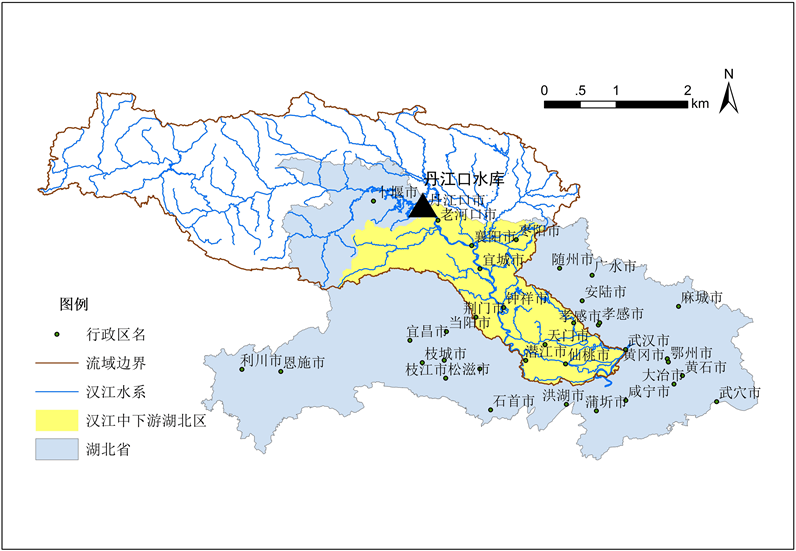Figure 1. Sketch map of study area and location in the middle and lower Han River basin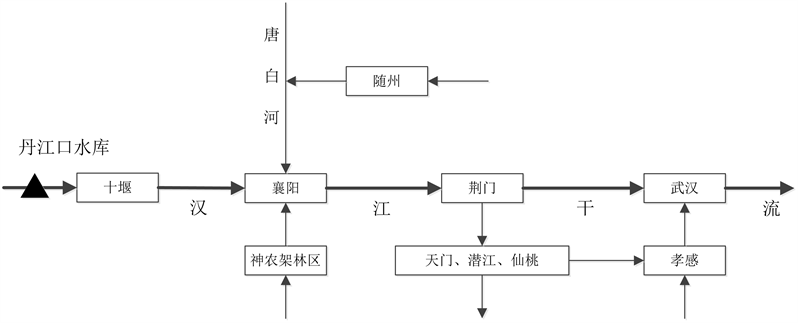Figure 2. Conceptual diagram for water resources system in the middle and lower Han River basinTable 1. Divisions for water resources allocation in the middle and lower Han River basinTable 2. Comparison of water consumptions in current year and planning year in the middle and lower Han River basin

${P}_{a}={\gamma }_{a}\underset{i=1}{\overset{m}{\sum }}{A}_{i}\left({Y}_{i}-{Y}_{oi}\right){P}_{i}-{C}_{a}/{W}_{a}$ (17)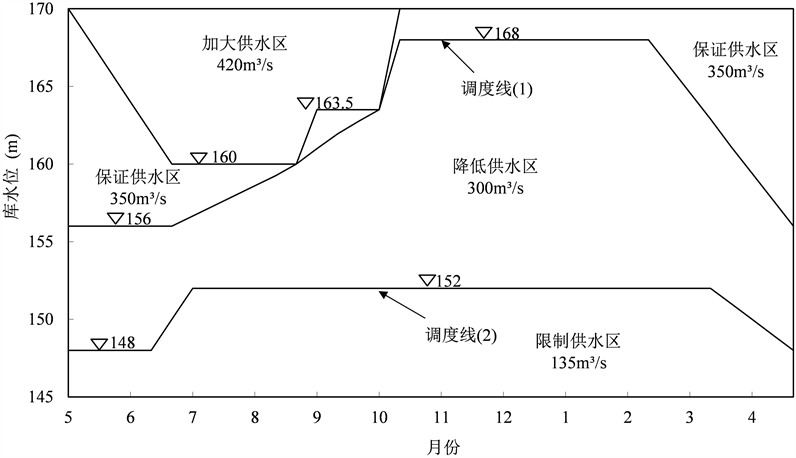Figure 3. Danjiangkou Reservoir operation chart in normal water supply operation periodTable 3. Percentage of lowest ecological water demand in the rivers

${P}_{i}=\frac{10000×{\gamma }_{i}}{{q}_{i}}-{C}_{i}$ (18)

4. 结果与讨论

4.1. 三步法求解结果及分析

HWP模型是让单个分区的效益最大化，据此求得各分区的配置水量。如模型1即代表进行水资源配置时，随州市取最大经济效益。从表4中数据分析可知，位于唐白河流域的分区，其配置效益受其它分区配水影响较大，而丹江口干流以下区域由于受到水库调蓄优化，配置效益不受其它分区的配水影响。

4.2. 不同频率配置结果及分析

2020规划水平年取农业灌溉需水频率分别为50%，75%和95%，采用各计算分区1956~2000年逐月长Table 4. Optimal benefit for each division in 2020 in the middle and lower Han River basin (Unit: 0.1 billion yuan)Table 5. Benefit ratio of each month calculated by the HRHWP modelTable 6. Optimal allocation water for each division in 2020 (50%) in the middle and lower Han River basinTable 7. Water resources supply and demand balance at different frequency in the middle and lower Han River basin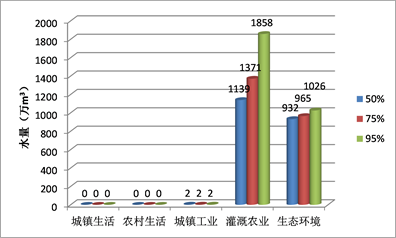(a) 随州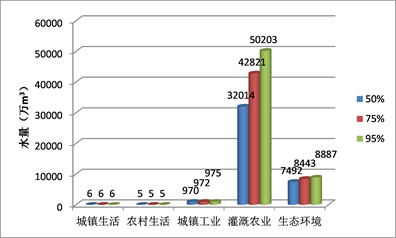(b) 襄阳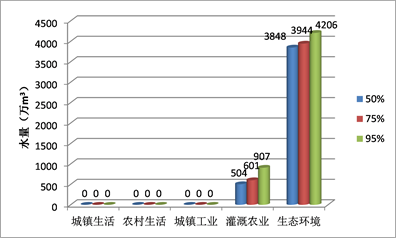(c) 神农架林区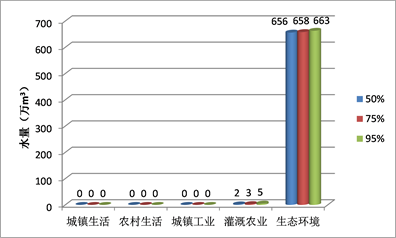(d) 十堰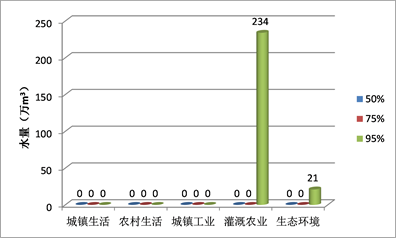(e) 荆门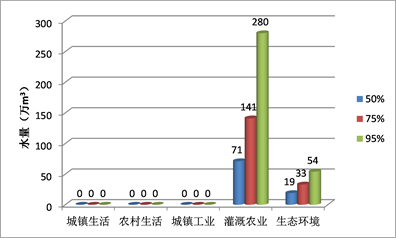(f) 天门潜江仙桃

Figure 4. Water shortage for each division in the middle and lower Han River basin

5. 结论

1) HWP模型结果表明，位于丹江口水库以上的分区，包括随州市和襄阳市，其配置效益受其它用水分区配水影响较大，而丹江口干流以下区域由于受水库调蓄优化，配置效益不受其他分区配水影响，全区总配置效益可达2150.55亿元。

2) 从HRHWP模型结果得到的效益比看出，由于夏季需水量最大，效益比值相对较小，而春、秋和冬季的效益比均超过0.9，表明夏季用水满足程度低于其它季节。

3) FWP模型结果表明，2020水平年在50%频率下，汉江中下游湖北区域河道外的总缺水量为3.47亿m3，其中农业和工业缺水量分别为3.37亿m3和972万m3，生活用水基本满足要求。

4) 当频率分别为50%、75%和95%时，2020年汉江中下游湖北地区缺水率分别为3.11%、3.69%和3.94%。其中，城镇和农村生活用水基本得到满足，而农业灌溉和河道内生态环境用水缺口较大。随着频率增高，需水缺口逐步增大。

5) 从三级水资源分区来看，由于缺少丹江口水库的调节，唐白河流域的缺水情况明显较丹江口以下干流地区严重，三种频率下总体缺水率分别达到24.84%，27.86%和29.75%。而丹江口以下地区总体缺水率分别仅为0.49%，0.57%和0.65%。

  冯耀龙, 韩文秀, 王宏江, 等. 面向可持续发展的区域水资源优化配置研究[J]. 系统工程理论与实践, 2003, 23(2): 133-138. FENG Yaolong, HAN Wenxiu, WANG Hongjiang, et al. Study on the sustainable development oriented optimal allocation to region water resources. Systems Engineering-Theory & Practice, 2003, 23(2): 133-138. (in Chinese)  王浩, 游进军. 中国水资源配置30年[J]. 水利学报, 2016, 47(3): 265-271. WANG Hao, YOU Jinjun. ZHENG Progress of water resources allocation during the past 30 years in China. Journal of Hydraulic Engineering, 2016, 47(3): 265-271. (in Chinese)  齐学斌, 黄仲冬, 乔冬梅, 等. 灌区水资源合理配置研究进展[J]. 水科学进展, 2015, 26(2): 287-295. QI Xuebin, HUANG Zhongdong, QIAO Dongmei, et al. Research advances on the reasonable water resources allocation in irrigation district. Advances in Water Science, 2015, 26(2): 287-295. (in Chinese)  LIU, D. D., GUO, S. L., SHAO, Q. X., et al. Assessing the effects of adaptation measures on optimal water resources allocation under varied water availability conditions. Journal of Hydrology, 2018, 556: 759-774. https://doi.org/10.1016/j.jhydrol.2017.12.002  邓铭江. 中国西北“水三线”空间格局与水资源配置方略[J]. 地理学报, 2018, 73(7): 1189-1203. DENG Mingjiang. “Three Water Lines” strategy: Its spatial patterns and effects on water resources allocation in northwest China. Acta Geographica Sinica, 2018, 73(7): 1189-1203. (in Chinese)  李建勋, 解建仓, 申静静, 等. 基于复杂性理论的水资源配置框架及方法体系[J]. 水电能源科学, 2017, 35(1): 21-24. LI Jianxun, XIE Jiancang, SHEN Jingjing, et al. Framework and method system of water resources allocation based on complexity theory. Water Resources and Power, 2017, 35(1): 21-24. (in Chinese)  吴丹, 吴凤平. 基于双层优化模型的流域初始二维水权耦合配置[J]. 中国人口•资源与环境, 2012, 22(10): 26-34. WU Dan, WU Fengping. The coupling allocation of initial two-dimensional water rights in basin based on the bi-hierarchy optimal model. China Population Resources and Environment, 2012, 22(10): 26-34. (in Chinese)  张妍, 郭萍, 张帆, 等. 基于评价的水资源优化模型适用性比较[J]. 排灌机械工程学报, 2018, 36(8): 1-5. ZHANG Yan, GUO Ping, ZHANG Fan, et al. Applicability comparison of water resources optimization model based on effect evaluation. Journal of Drainage and Irrigation Machinery Engineering, 2018, 36(8): 1-5. (in Chinese)  黄显峰, 邵东国, 顾文权, 等. 基于多目标混沌优化算法的水资源配置研究[J]. 水利学报, 2008, 39(2): 183-188. HUANG Xianfeng, SHAO Dongguo, GU Wenquan, et al. Optimal water resources deployment based on multi-objective chaotic optimization algorithm. Journal of Hydraulic Engineering, 2008, 39(2): 183-188. (in Chinese)  何国华, 解建仓, 汪妮, 等. 基于模拟退火遗传算法的水资源优化配置研究[J]. 西北农林科技大学学报(自然科学版), 2016, 44(6): 196-202. HE Guohua, XIE Jiancang, WANG Ni, et al. Optimal allocation of water resources based on simulated annealing-genetic algorithm. Journal of Northwest A&F University (Natural Science Edition), 2016, 44(6): 196-202. (in Chinese)  李维乾, 解建仓, 李建勋, 等. 基于灰色理论及改进类电磁学算法的水资源配置[J]. 水利学报, 2012, 43(12): 1447-1456+1463. LI Weiqian, XIE Jiancang, LI Jianxun, et al. Water resources allocation based on the grey theory and the improved electromagnetism-like algorithm. Journal of Hydraulic Engineering, 2012, 43(12): 1447-1456+1463. (in Chinese)  沙金霞. 改进鲸鱼算法在多目标水资源优化配置中的应用[J]. 水利水电技术, 2018, 49(4): 18-26. SHA Jinxia. Application of ameliorative whale optimization algorithm to optimal allocation of multi-objective water resources. Water Resources and Hydropower Engineering, 2018, 49(4): 18-26. (in Chinese)  王梟涵, 丁文静, 赖学方. 基于蝙蝠优化算法的水资源配置[J]. 西安工程大学学报, 2016, 30(2): 268-275. WANG Xiaohan, DING Wenjing, and LAI Xuefang. Optimal allocation of water resources based on bat algorithm. Journal of Xi’an Polytechnic University, 2016, 30(2): 268-275. (in Chinese)  刘德地, 郭生练, 郭海晋, 等. 实施最严格水资源管理制度面临的技术问题与挑战[J]. 水资源研究, 2014(3): 179-188. LIU Dedi, GUO Shenglian, GUO Haijin, et al. Technique controversies and challenges of applying the strictest water resources control system. Journal of Water Resources Research, 2014(3): 179-188. (in Chinese)  田晶, 郭生练, 刘德地, 等. 汉江中下游地区水资源多目标优化配置[J]. 水资源研究, 2018, 7(3): 223-235. TIAN Jing, GUO Shenglian, LIU Dedi, et al. Multi-objective optimal allocation of water resources in the middle and lower reaches of Hanjiang River basin. Journal of Water Resources Research, 2018, 7(3): 223-235. (in Chi-nese)  ROOZBAHANI, R., ABBASI, B., SCHREIDER, S., et al. A multi-objective approach for transboundary river water allocation. Water Resources Management, 2014, 15(28): 5447-5463.  甘泓, 汪林, 倪红珍, 等. 水经济价值计算方法评价研究[J]. 水利学报, 2008, 39(11): 1160-1166. GAN Hong, WANG Lin, NI Hongzhen, et al. Assessment on methods for calculating economic value of water. Journal of Hydraulic Engineering, 2008, 39(11): 1160-1166. (in Chinese)  孙静, 阮本清, 张春玲. 新安江流域上游地区水资源价值计算与分析[J]. 中国水利水电科学研究院学报, 2007, 5(2): 121-124. SUN Jing, RUAN Benqing, and ZHANG Chunling. Calculation and analysis of water resources value in upper reaches of Xinan River. Journal of China Institute of Water Resources and Hydropower Research, 2007, 5(2): 121-124. (in Chinese)  沈佩君, 关庆滔. 对湖北江汉平原水稻棉花灌溉效益分摊比例的分析[J]. 水利水电技术, 1983(8): 15-20. SHEN Peijun, GUAN Qingtao. Analysis on the proportion of irrigation benefit of rice and cotton in Hubei Jianghan Plain. Water Resources and Hydropower Engineering, 1983(8): 15-20. (in Chinese)